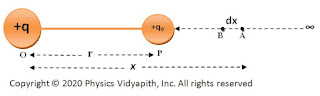### Electric Potential and Derivation of Electric Potential at a Point due to Point Charged Particle

Electric Potential:

When a test-charged particle is brought from infinity to a point in the electric field then the work done per unit test charge particle is called electric potential. It is represented by $V$. It is a scalar quantity.

Let's consider a test-charged particle $q_{0}$ bring from infinity to at a point $P$ in the electric field. If the work done by test charged particle is $W$ then electric potential →

 $V=\frac{W}{q_{0}}$

Unit of Electric Potential: $Joul/Coulomb$  OR  $N-m/Ampere-sec$

In MKS: $Kg-m^{2}-Ampere^{-1}-sec^{-3}$

Dimension of Electric potential: $[ML^{2}A^{-1}T^{-3}]$

The electric potential at a point due to point charged particle:

Let us consider, a source point charge $+q$ is placed in air and vacuum at point $O$.Let's take a point $P$ at distance $r$ from the source point charged particle. Here the test-charged particle $+q_{0}$ is brought from infinity to point $P$.If the test-charged particle moves a very small distance $dx$ from point $A$ to $B$ against the electrostatic force. So electrostatic force at point $A$ which is placed at a distance $x$ from point $O$ →

$F=\frac{1}{4\pi\epsilon{0}} \frac{qq_{0}}{x^{2}} \qquad(1)$Electric potential due to point charge
The work is done against the electrostatic force $\overrightarrow{F}$ to move small distance $dx$ from point $A$ to Point $B$

$dW=\overrightarrow{F}\: \overrightarrow{dx}$

$dW=F\: dx \: cos 180^{\circ}$

Here the angle between the electrostatic force and displacement is $180^{\circ}$. So work done

$dW=- F\: dx$

$dW=-\frac{1}{4\pi\epsilon{0}} \frac{qq_{0}}{x^{2}}\:dx \qquad \left\{ from\: equation \: (1) \right\}$

The total work is done in moving the charge $q_{0}$ from infinity to the point $P$ will be

$W=-\int_{0}^{W}{dW}$

Here negative sign shows that the work done from infinity to at point $P$ is stored in the form of potential energy between the charges.

$W=-\int_{\infty}^{r}{\frac{1}{4\pi\epsilon{0}} \frac{qq_{0}}{x^{2}}\:dx }$

$W=-\frac{qq_{0}}{4\pi\epsilon{0}} \int_{\infty}^{r}{\frac{dx}{x^{2}} }$

$W=-\frac{qq_{0}}{4\pi\epsilon{0}} \left [\frac{-1}{x} \right ]_{\infty}^{r}$

$W=-\frac{qq_{0}}{4\pi\epsilon{0}} \left [-\frac{1}{r}-\frac{-1}{\infty} \right ]$

$W=\frac{qq_{0}}{4\pi\epsilon{0}} \left [\frac{1}{r} \right ]$

$W=\frac{1}{4\pi\epsilon{0}} \left [\frac{qq_{0}}{r} \right ]$

Hence, the work is done to move a unit test charge from infinity to the point $P$, or the electric potential at point $P$ is →

$V=\frac{W}{q_{0}}$

 $V=\frac{1}{4\pi\epsilon{0}} \frac{q}{r}$